# Making a Multiplayer FPS in Unity

### All free Making a Multiplayer FPS in Unity. You will find here all free Video Lectures on Programming

Programming Book have 32 video Lectures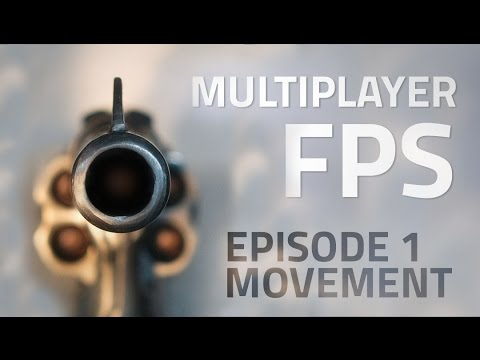Making a Multiplayer FPS in Unity (EP. 1) – uNet Tutorial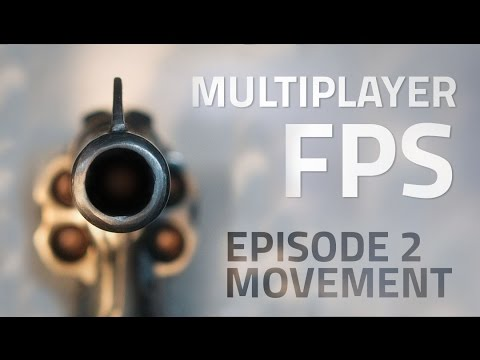Making a Multiplayer FPS in Unity (E02. Movement) – uNet Tutorial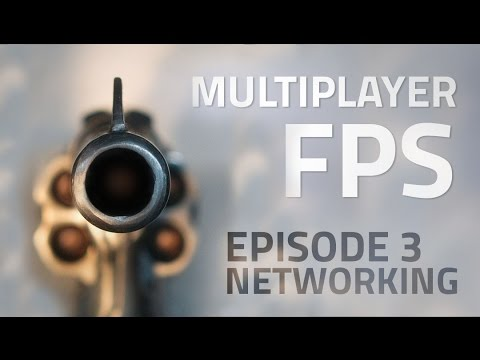Making a Multiplayer FPS in Unity (E03. Networking Intro) – uNet Tutorial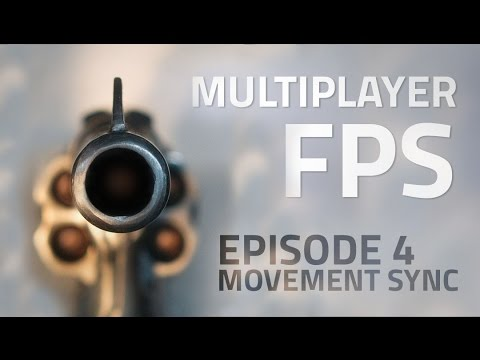Making a Multiplayer FPS in Unity (E04. Syncing Movement) – uNet Tutorial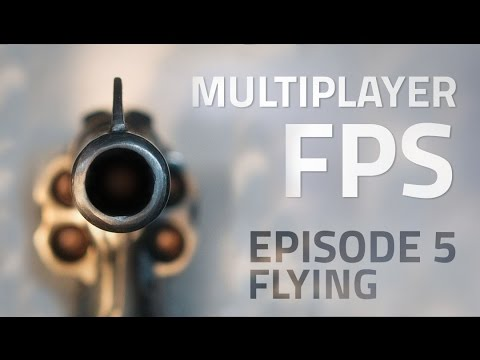Making a Multiplayer FPS in Unity (E05. Flying) – uNet Tutorial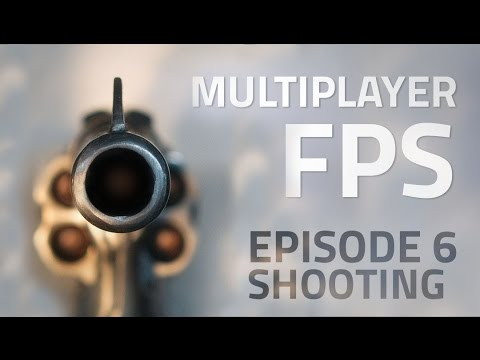Making a Multiplayer FPS in Unity (E06. Shooting) – uNet TutorialMaking a Multiplayer FPS in Unity (E07. Damage) – uNet Tutorial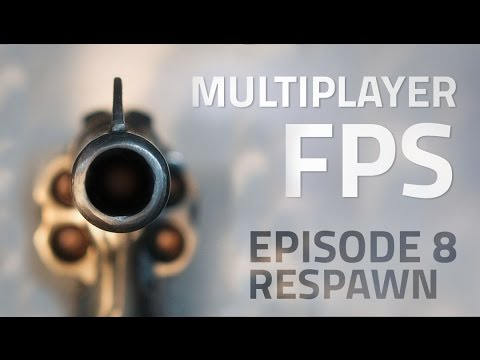Making a Multiplayer FPS in Unity (E08. Respawn) – uNet Tutorial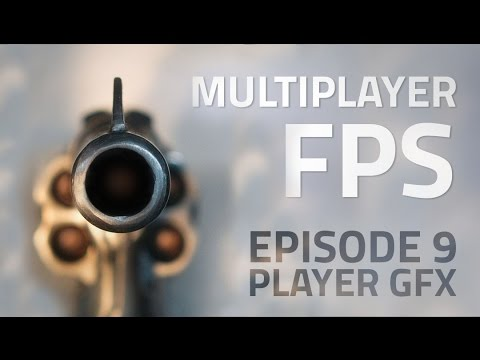Making a Multiplayer FPS in Unity (E09. Player Model) – uNet Tutorial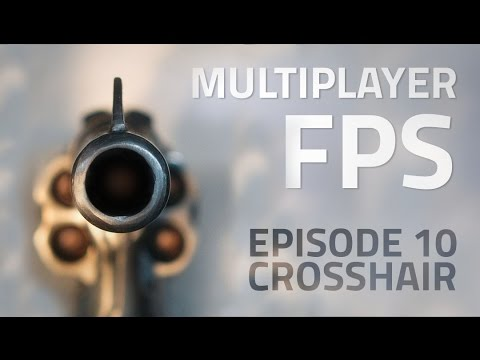Making a Multiplayer FPS in Unity (E10. Crosshair) – uNet Tutorial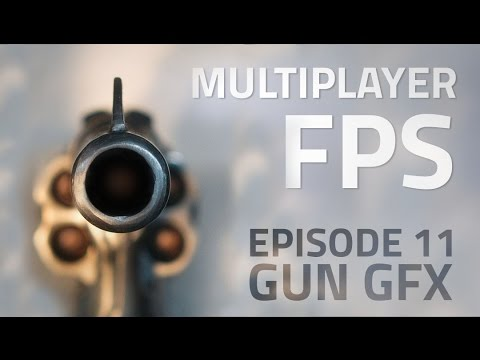Making a Multiplayer FPS in Unity (E11. Gun GFX) – uNet Tutorial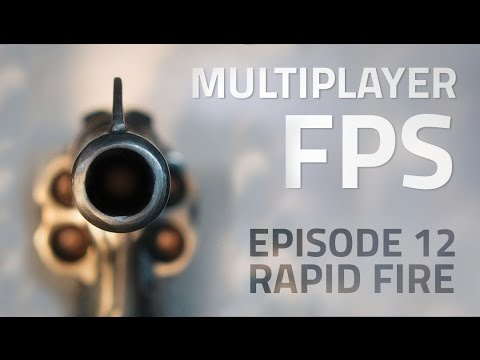Making a Multiplayer FPS in Unity (E12. Rapid Fire) – uNet Tutorial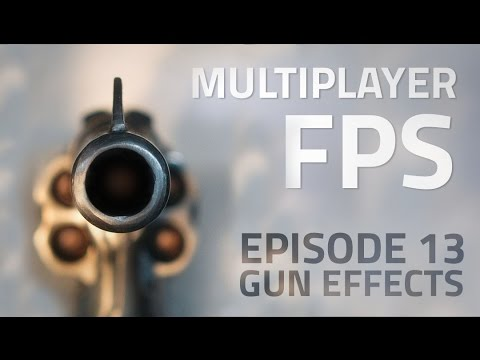Making a Multiplayer FPS in Unity (E13. Muzzle Flash) – uNet Tutorial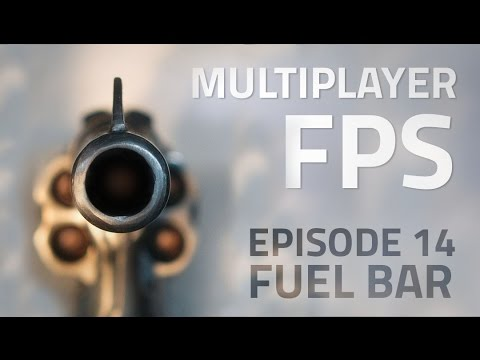Making a Multiplayer FPS in Unity (E14. Fuel) – uNet Tutorial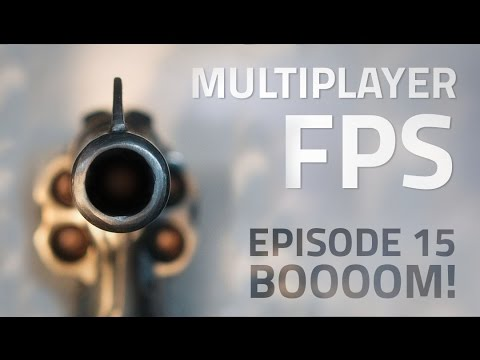Making a Multiplayer FPS in Unity (E15. Explosions) – uNet Tutorial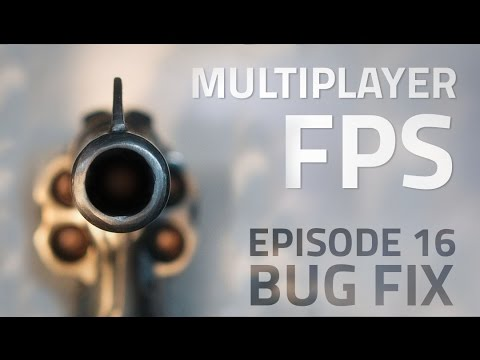Making a Multiplayer FPS in Unity (E16. Bug Fixing) – uNet Tutorial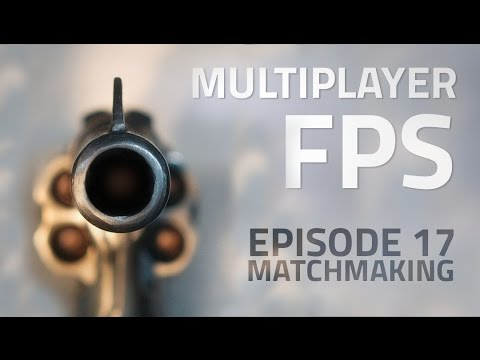Making a Multiplayer FPS in Unity (E17. Matchmaking) – uNet Tutorial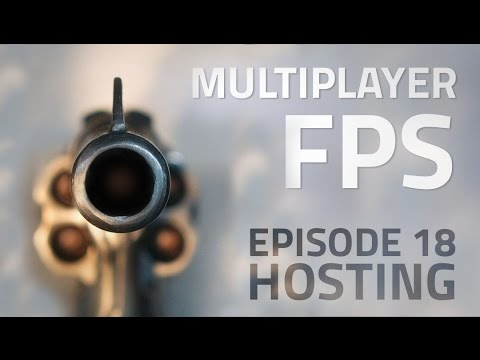Making a Multiplayer FPS in Unity (E18. Hosting) – uNet Tutorial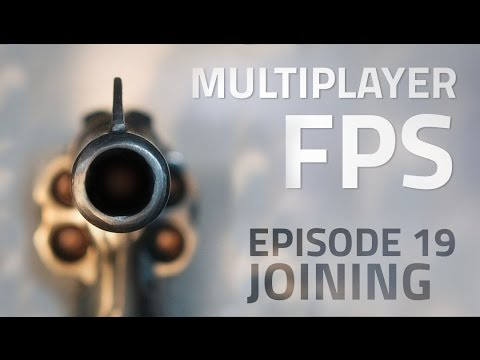Making a Multiplayer FPS in Unity (E19. Joining 1/2) – uNet Tutorial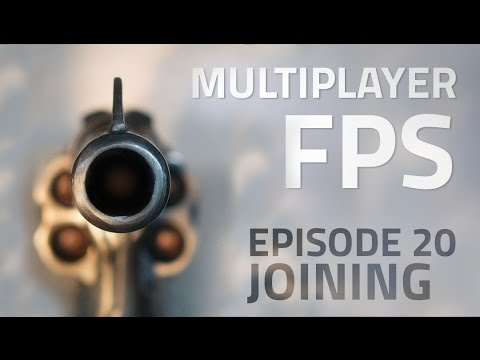Making a Multiplayer FPS in Unity (E20. Joining 2/2) – uNet Tutorial